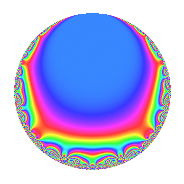# Properties

 Label 55.2.a.bLevel 55 Weight 2 Character orbit 55.a Self dual Yes Analytic conductor 0.439 Analytic rank 0 Dimension 2 CM No Inner twists 1

# Related objects

## Newspace parameters

 Level: $$N$$ = $$55 = 5 \cdot 11$$ Weight: $$k$$ = $$2$$ Character orbit: $$[\chi]$$ = 55.a (trivial)

## Newform invariants

 Self dual: Yes Analytic conductor: $$0.439177211117$$ Analytic rank: $$0$$ Dimension: $$2$$ Coefficient field: $$\Q(\sqrt{2})$$ Coefficient ring: $$\Z[a_1, a_2]$$ Coefficient ring index: $$1$$ Fricke sign: $$-1$$ Sato-Tate group: $\mathrm{SU}(2)$

## $q$-expansion

Coefficients of the $$q$$-expansion are expressed in terms of $$\beta = \sqrt{2}$$. We also show the integral $$q$$-expansion of the trace form.

 $$f(q)$$ $$=$$ $$q + ( 1 + \beta ) q^{2} -2 \beta q^{3} + ( 1 + 2 \beta ) q^{4} - q^{5} + ( -4 - 2 \beta ) q^{6} -2 q^{7} + ( 3 + \beta ) q^{8} + 5 q^{9} +O(q^{10})$$ $$q + ( 1 + \beta ) q^{2} -2 \beta q^{3} + ( 1 + 2 \beta ) q^{4} - q^{5} + ( -4 - 2 \beta ) q^{6} -2 q^{7} + ( 3 + \beta ) q^{8} + 5 q^{9} + ( -1 - \beta ) q^{10} + q^{11} + ( -8 - 2 \beta ) q^{12} + ( -4 + 2 \beta ) q^{13} + ( -2 - 2 \beta ) q^{14} + 2 \beta q^{15} + 3 q^{16} + ( 4 + 2 \beta ) q^{17} + ( 5 + 5 \beta ) q^{18} + ( -1 - 2 \beta ) q^{20} + 4 \beta q^{21} + ( 1 + \beta ) q^{22} -2 \beta q^{23} + ( -4 - 6 \beta ) q^{24} + q^{25} -2 \beta q^{26} -4 \beta q^{27} + ( -2 - 4 \beta ) q^{28} + ( 2 - 4 \beta ) q^{29} + ( 4 + 2 \beta ) q^{30} + ( -3 + \beta ) q^{32} -2 \beta q^{33} + ( 8 + 6 \beta ) q^{34} + 2 q^{35} + ( 5 + 10 \beta ) q^{36} + ( -2 - 4 \beta ) q^{37} + ( -8 + 8 \beta ) q^{39} + ( -3 - \beta ) q^{40} + 6 q^{41} + ( 8 + 4 \beta ) q^{42} -6 q^{43} + ( 1 + 2 \beta ) q^{44} -5 q^{45} + ( -4 - 2 \beta ) q^{46} + 2 \beta q^{47} -6 \beta q^{48} -3 q^{49} + ( 1 + \beta ) q^{50} + ( -8 - 8 \beta ) q^{51} + ( 4 - 6 \beta ) q^{52} + ( 6 + 4 \beta ) q^{53} + ( -8 - 4 \beta ) q^{54} - q^{55} + ( -6 - 2 \beta ) q^{56} + ( -6 - 2 \beta ) q^{58} + ( -4 + 4 \beta ) q^{59} + ( 8 + 2 \beta ) q^{60} + ( 2 - 8 \beta ) q^{61} -10 q^{63} + ( -7 - 2 \beta ) q^{64} + ( 4 - 2 \beta ) q^{65} + ( -4 - 2 \beta ) q^{66} + ( 4 + 6 \beta ) q^{67} + ( 12 + 10 \beta ) q^{68} + 8 q^{69} + ( 2 + 2 \beta ) q^{70} + 8 \beta q^{71} + ( 15 + 5 \beta ) q^{72} + ( -4 + 2 \beta ) q^{73} + ( -10 - 6 \beta ) q^{74} -2 \beta q^{75} -2 q^{77} + 8 q^{78} + 4 q^{79} -3 q^{80} + q^{81} + ( 6 + 6 \beta ) q^{82} -6 q^{83} + ( 16 + 4 \beta ) q^{84} + ( -4 - 2 \beta ) q^{85} + ( -6 - 6 \beta ) q^{86} + ( 16 - 4 \beta ) q^{87} + ( 3 + \beta ) q^{88} + ( -2 - 8 \beta ) q^{89} + ( -5 - 5 \beta ) q^{90} + ( 8 - 4 \beta ) q^{91} + ( -8 - 2 \beta ) q^{92} + ( 4 + 2 \beta ) q^{94} + ( -4 + 6 \beta ) q^{96} + ( -2 + 4 \beta ) q^{97} + ( -3 - 3 \beta ) q^{98} + 5 q^{99} +O(q^{100})$$ $$\operatorname{Tr}(f)(q)$$ $$=$$ $$2q + 2q^{2} + 2q^{4} - 2q^{5} - 8q^{6} - 4q^{7} + 6q^{8} + 10q^{9} + O(q^{10})$$ $$2q + 2q^{2} + 2q^{4} - 2q^{5} - 8q^{6} - 4q^{7} + 6q^{8} + 10q^{9} - 2q^{10} + 2q^{11} - 16q^{12} - 8q^{13} - 4q^{14} + 6q^{16} + 8q^{17} + 10q^{18} - 2q^{20} + 2q^{22} - 8q^{24} + 2q^{25} - 4q^{28} + 4q^{29} + 8q^{30} - 6q^{32} + 16q^{34} + 4q^{35} + 10q^{36} - 4q^{37} - 16q^{39} - 6q^{40} + 12q^{41} + 16q^{42} - 12q^{43} + 2q^{44} - 10q^{45} - 8q^{46} - 6q^{49} + 2q^{50} - 16q^{51} + 8q^{52} + 12q^{53} - 16q^{54} - 2q^{55} - 12q^{56} - 12q^{58} - 8q^{59} + 16q^{60} + 4q^{61} - 20q^{63} - 14q^{64} + 8q^{65} - 8q^{66} + 8q^{67} + 24q^{68} + 16q^{69} + 4q^{70} + 30q^{72} - 8q^{73} - 20q^{74} - 4q^{77} + 16q^{78} + 8q^{79} - 6q^{80} + 2q^{81} + 12q^{82} - 12q^{83} + 32q^{84} - 8q^{85} - 12q^{86} + 32q^{87} + 6q^{88} - 4q^{89} - 10q^{90} + 16q^{91} - 16q^{92} + 8q^{94} - 8q^{96} - 4q^{97} - 6q^{98} + 10q^{99} + O(q^{100})$$

## Embeddings

For each embedding $$\iota_m$$ of the coefficient field, the values $$\iota_m(a_n)$$ are shown below.

For more information on an embedded modular form you can click on its label.

Label $$\iota_m(\nu)$$ $$a_{2}$$ $$a_{3}$$ $$a_{4}$$ $$a_{5}$$ $$a_{6}$$ $$a_{7}$$ $$a_{8}$$ $$a_{9}$$ $$a_{10}$$
1.1
 −1.41421 1.41421
−0.414214 2.82843 −1.82843 −1.00000 −1.17157 −2.00000 1.58579 5.00000 0.414214
1.2 2.41421 −2.82843 3.82843 −1.00000 −6.82843 −2.00000 4.41421 5.00000 −2.41421
 $$n$$: e.g. 2-40 or 990-1000 Significant digits: Format: Complex embeddings Normalized embeddings Satake parameters Satake angles

## Inner twists

This newform does not admit any (nontrivial) inner twists.

## Atkin-Lehner signs

$$p$$ Sign
$$5$$ $$1$$
$$11$$ $$-1$$

## Hecke kernels

This newform can be constructed as the kernel of the linear operator $$T_{2}^{2} - 2 T_{2} - 1$$ acting on $$S_{2}^{\mathrm{new}}(\Gamma_0(55))$$.